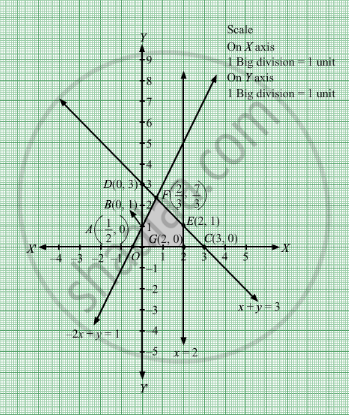# Maximize Z = X + Y Subject to − 2 X + Y ≤ 1 X ≤ 2 X + Y ≤ 3 X , Y ≥ 0 - Mathematics

Maximize Z = x + y
Subject to

$- 2x + y \leq 1$
$x \leq 2$
$x + y \leq 3$
$x, y \geq 0$

#### Solution

We need to maximize Z = x + y
First, we will convert the given inequations into equations, we obtain the following equations:
2x + y = 1, x = 2, x + y = 3, x = 0 and y = 0.
The line 2x + y = 1 meets the coordinate axis at $A\left( \frac{- 1}{2}, 0 \right)$ and B(0, 1). Join these points to obtain the line 2x + y = 1 .
Clearly, (0, 0) satisfies the inequation 2x + y ≤ 1. So, the region in xy-plane that contains the origin represents the solution set of the given equation.
x = 2 is the line passing through (2, 0) and parallel to the Y axis.The region below the line x = 2 will satisfy the given inequation.
The line x + y = 3 meets the coordinate axis at C(3, 0) and D(0, 3). Join these points to obtain the line x + y = 3.
Clearly, (0, 0) satisfies the inequation x + ≤ 3. So, the region in xy-plane that contains the origin represents the solution set of the given equation.
Region represented by x ≥ 0 and y ≥ 0:
Since, every point in the first quadrant satisfies these inequations. So, the first quadrant is the region represented by the inequations.
These lines are drawn using a suitable scale.The corner points of the feasible region are O(0, 0), $G\left( 2, 0 \right)$ ,$E\left( 2, 1 \right)$ and $F\left( \frac{2}{3}, \frac{7}{3} \right)$

The values of Z at these corner points are as follows.

 Corner point Z = x + y O(0, 0) 0 +  0 = 0 $C\left( 2, 0 \right)$ 2 + 0 = 2 $E\left( 2, 1 \right)$ 2 +1 = 3 $F\left( \frac{2}{3}, \frac{7}{3} \right)$ $\frac{2}{3} + \frac{7}{3} = \frac{9}{3} = 3$

We see that the maximum value of the objective function Z is 3 which is at $E\left( 2, 1 \right)$ and $F\left( \frac{2}{3}, \frac{7}{3} \right)$

Thus, the optimal value of Z is 3.

Concept: Graphical Method of Solving Linear Programming Problems
Is there an error in this question or solution?

#### APPEARS IN

RD Sharma Class 12 Maths
Chapter 30 Linear programming
Exercise 30.2 | Q 19 | Page 33